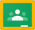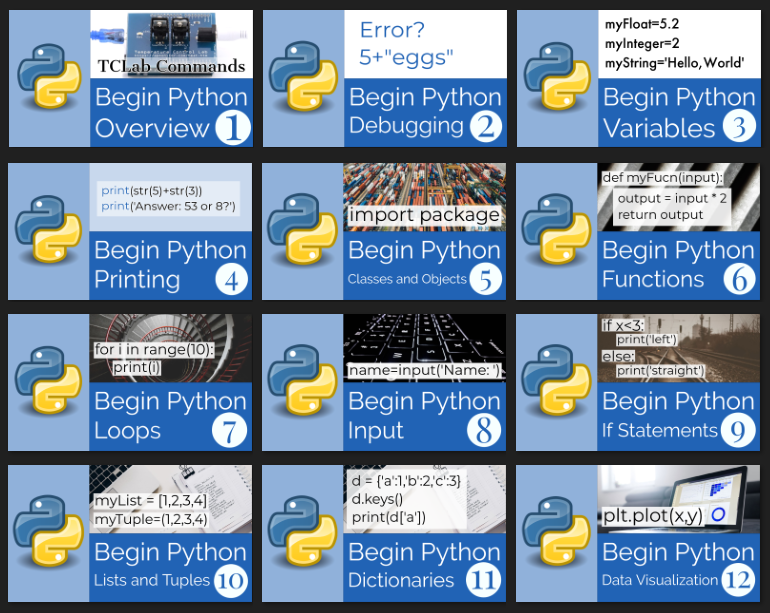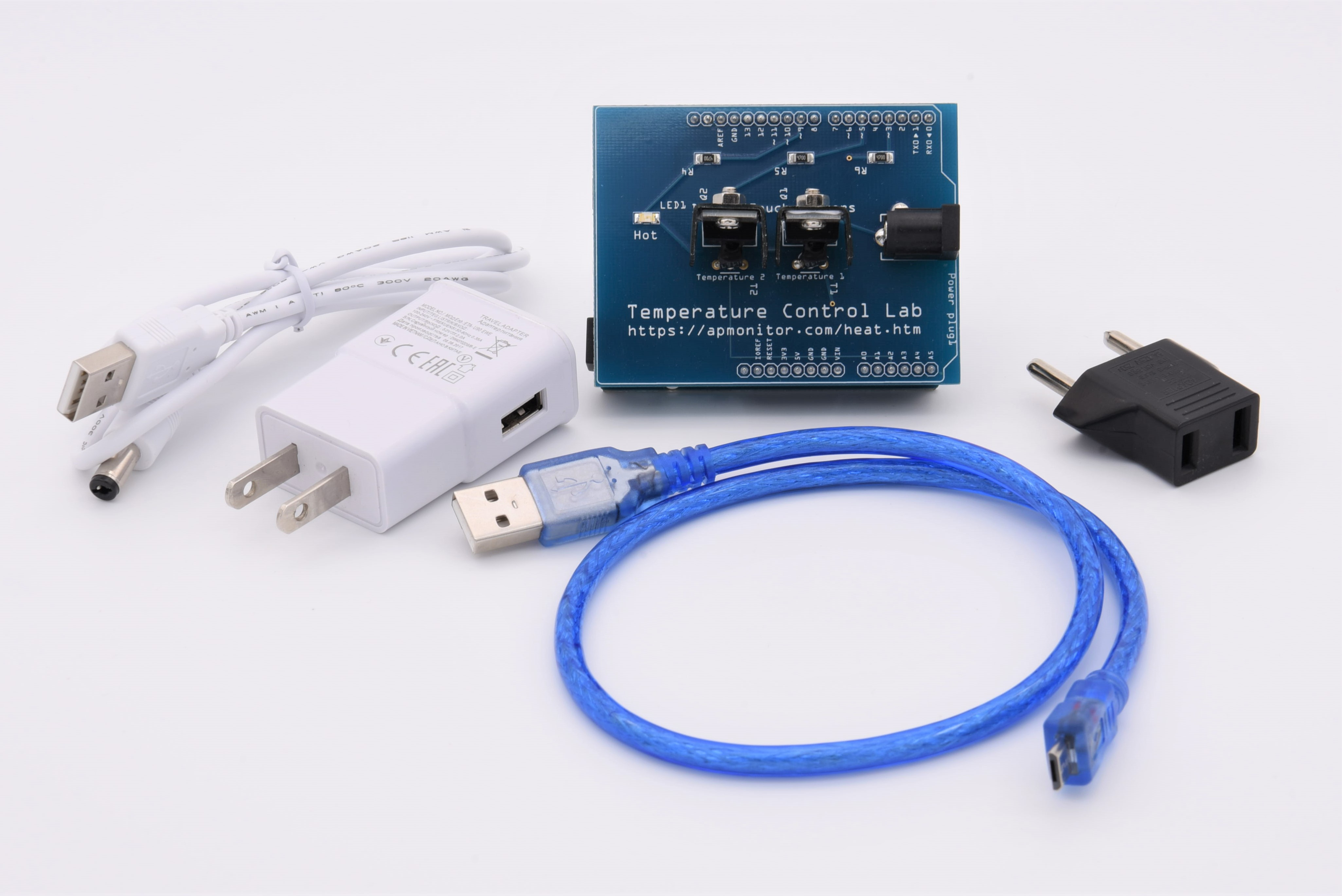## Begin Python with TCLab

The Begin Python with TCLab is an introduction and review of basic Python programming with 12 lessons that can be completed in 2-3 hours (15-20 minutes each).Begin Python 🐍 with TCLab (GitHub)Begin Python 🐍 in Google ClassroomELA270: Introduction to Python 🐍 with AIChE Academy

The course is designed to start from no programming experience and guide a self-paced learner through the basics of Python. The 12 modules are IPython notebooks that are run from a Jupyter Notebook. The tutorials start with how to install Anaconda and use the hands-on lab kit. The exercises are interactive and there are solution videos for each module for those who need additional help.After completing this course, there are next courses that build upon the basic Python programming experience.

#### Temperature Control Lab

The final project is a review of all course material with real data from temperature sensors in the Temperature Control Lab (TCLab). The temperatures are adjusted with heaters that are adjusted with the TCLab. This lab hardware is also used in the Process Dynamics and Control Course and the Dynamic Optimization Course.#### TCLab Digital Twin

If no hardware is available, use TCLabModel() in the place of TCLab(). The emulator (digital twin) can be run faster than real-time as shown below:

import tclab
import numpy as np

tclab_hardware = False
if tclab_hardware:
mlab = tclab.TCLab      # Physical hardware
else:
speedup = 100           # Emulator (digital twin) speed-up
mlab = tclab.setup(connected=False, speedup=speedup)

n = 500
tm = np.linspace(0,2*n,n+1)

# Connect to TCLab
with mlab() as lab:
# set heater values
lab.Q1(70)
lab.Q2(20)
for t in tclab.clock(tm[-1]+1, 2):
print('Time: ' + str(t) + \
' T1: ' + str(round(lab.T1,2)) + \
' T2: ' + str(round(lab.T2,2)))

Change tclab_hardware from False to True to use the physical hardware.